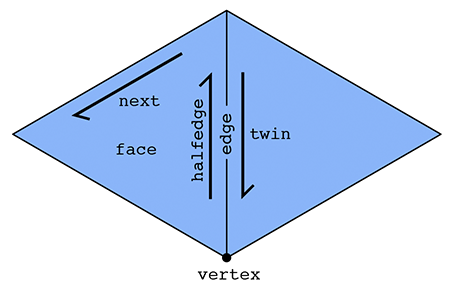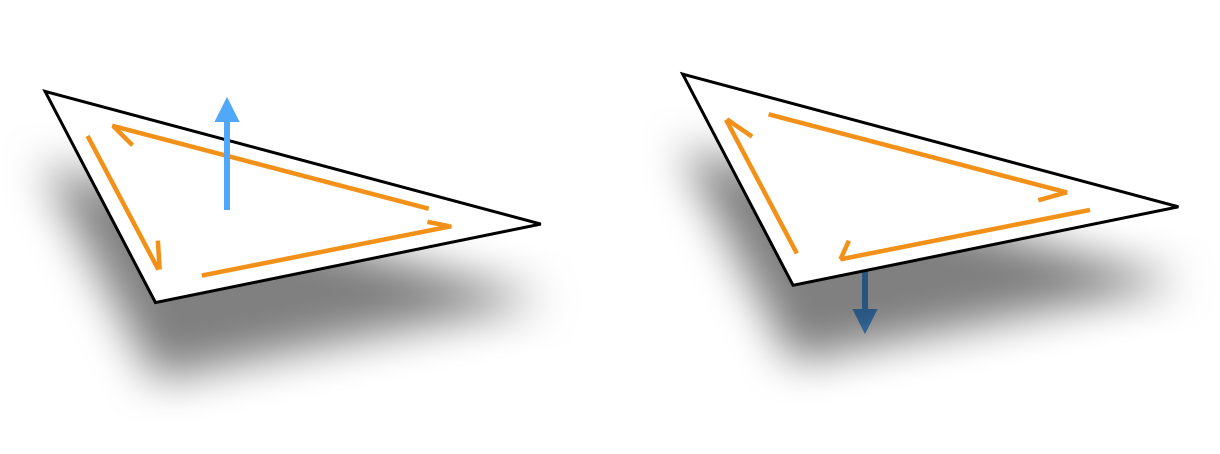# Halfedge meshes

The halfedge mesh is a powerful and flexible data structure for representing oriented, manifold polygonal meshes, and is the core data structure in geometry-central.

The halfedge mesh has several key advantages over other data structures, most notably that all adjacent-neighborhood traversals can be implemented in constant time, without the use of any variably-sized neighbor lists. Furthermore, common mutation operations like edge splits and vertex insertions can be performed in constant time. This halfedge mesh implementation furthermore stores all elements in contiguous buffers of memory, which makes it fast (see internals for implementation details).

As the name suggests, the primary type in a halfedge mesh is a halfedge, in addition to the usual vertex, edge and face types. A halfedge is a directed edge incident on a face, as shown below. Two halfedges, oriented in opposite directions, make up each edge in the mesh. Each halfedge has relationships with five adjacent elements:

• Halfedge::twin() the other halfedge across the incident edge
• Halfedge::next() the next halfedge in clockwise order around the incident face
• Halfedge::vertex() the vertex at the tail (back) of the halfedge
• Halfedge::edge() the incident edge
• Halfedge::face() the incident faceEach vertex, edge, and face need just one relationship:

• Vertex::halfedge() any of the incident halfedges (which point outward from the vertex)
• Edge::halfedge() any of the incident halfedges
• Face::halfedge() any of the incident halfedges

In fact, this fixed set of relationships is sufficient to implement pretty much any local traversal. Geometry central provides a wide range of convience iterators which wrap these relationships to traverse neighborhoods, such as the example below.

for(Edge e : vertex.adjacentEdges()) {
// do science
}


Notice that the lightweight Halfedge (etc) types serve simply as logical references, or “handles” to a mesh element. Deleting one of these handles does not delete the underlying element, and one may have multiple handles to the same element Vertex a; Vertex b; a == b;.

## Manifold, Oriented Surfaces

The basic halfedge mesh imposes two requirements: manifoldness and orientability.

Manifoldness means that our surface must locally look like a plane in any neighborhood. This disallows structures such as three faces meeting at an edge, or two cones of faces meeting at a single vertex like an hourglass.

Furthermore the halfedge mesh implies a combinatorial orientation of the surface, indicated by the clockwise ordering of halfedges around each face (see figure below). Because the halfedge mesh implies an orientation, it cannot represent non-orientable surfaces, like a Klein bottle.These properties are invariants which always hold for any meaningful halfedge mesh; in practice we check them during construction and ensure that all operations preserve them.

Note that our halfedge mesh does not require that faces be triangles or quads; arbitrary faces with degree >= 3 are supported, and faces of different degree may be intermingled. However, many operations are only defined for triangle meshes and will throw errors if invoked on other meshes.

## Basic API

### Constructors

HalfedgeMesh(const std::vector<std::vector<size_t>>& polygons, bool verbose = false)

Constructs a halfedge mesh from a face-index list.

• polygons a list of faces, each holding the indices of the vertices incident on that face, zero-indexed and in counter-clockwise order.
• verbose if true, prints some statistics to std::cout during construction.

### Element counts

size_t HalfedgeMesh::nVertices()

Returns the number of vertices.

size_t HalfedgeMesh::nInteriorVertices()

Returns the number of vertices not incident on the boundary.

size_t HalfedgeMesh::nBoundaryVertices()

Returns the number of vertices incident on the boundary.

size_t HalfedgeMesh::nEdges()

Returns the number of edges.

size_t HalfedgeMesh::nFaces()

Returns the number of faces in the mesh.

size_t HalfedgeMesh::nHalfedges()

Returns the number of halfedges, including both interior halfedges and any exterior halfedges incident on boundary loops. Always exactly twice the number of edges.

size_t HalfedgeMesh::nInterioHalfedges()

Returns the number of interior halfedges, which are incident on faces of the mesh. Always equal to the sum of the number of sides of all faces.

size_t HalfedgeMesh::nExteriorHalfedges()

Returns the number of exterior halfedges, which are opposite boundary faces.

size_t HalfedgeMesh::nBoundaryLoops()

Returns the number of distinct boundary loops in the mesh, each identified as an fictional face closing a boundary loop in the mesh.

### Properties

bool HalfedgeMesh::hasBoundary()

Returns true if the mesh has boundary, that is if it is not closed.

Complexity $\mathcal{O}(1)$.

int HalfedgeMesh::eulerCharacteristic()

Returns the Euler characteristic of the surface. Computed in O(1) from element counts.

Note: always computed by naively applying Euler’s polyhedron formula, which might not do what you want in the case of multiple-connected components.

int HalfedgeMesh::genus()

Returns the genus of the surface. Computed in O(1) from element counts.

Note: always computed by naively applying Euler’s polyhedron formula, which might not do what you want in the case of multiple connected components.

bool HalfedgeMesh::isTriangular()

Returns true if all faces in the mesh have 3 sides.

Complexity $\mathcal{O}(n)$, do not call in a tight loop.

size_t HalfedgeMesh::nConnectedComponents()

Returns the number of distinct connected components of the mesh.

Complexity $\mathcal{O}(n)$, do not call in a tight loop.

### Utility functions

std::unique_ptr<Halfedgemesh> copy()

Constructs a copy of the mesh.